# SAT II Physics : Capacitors

## Example Questions

### Example Question #1 : Capacitors

A capacitor with capacitanceis constructed by putting a thin piece of cardboard between two copper plates, then each plate is connected to a battery. If the copper plates are cut in half then what is the effect on the capacitance of the circuit?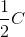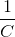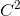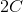Explanation:

Capacitance of a circuit is defined by the equation: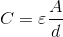Whereis the capacitance,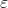is a constant of nature,is the area of the capacitor, andis the distance between the two plates

Since the metal plates are cut in half, the area is halved. We can substitute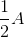in forThe result is that the capacitance is half the original quantity.